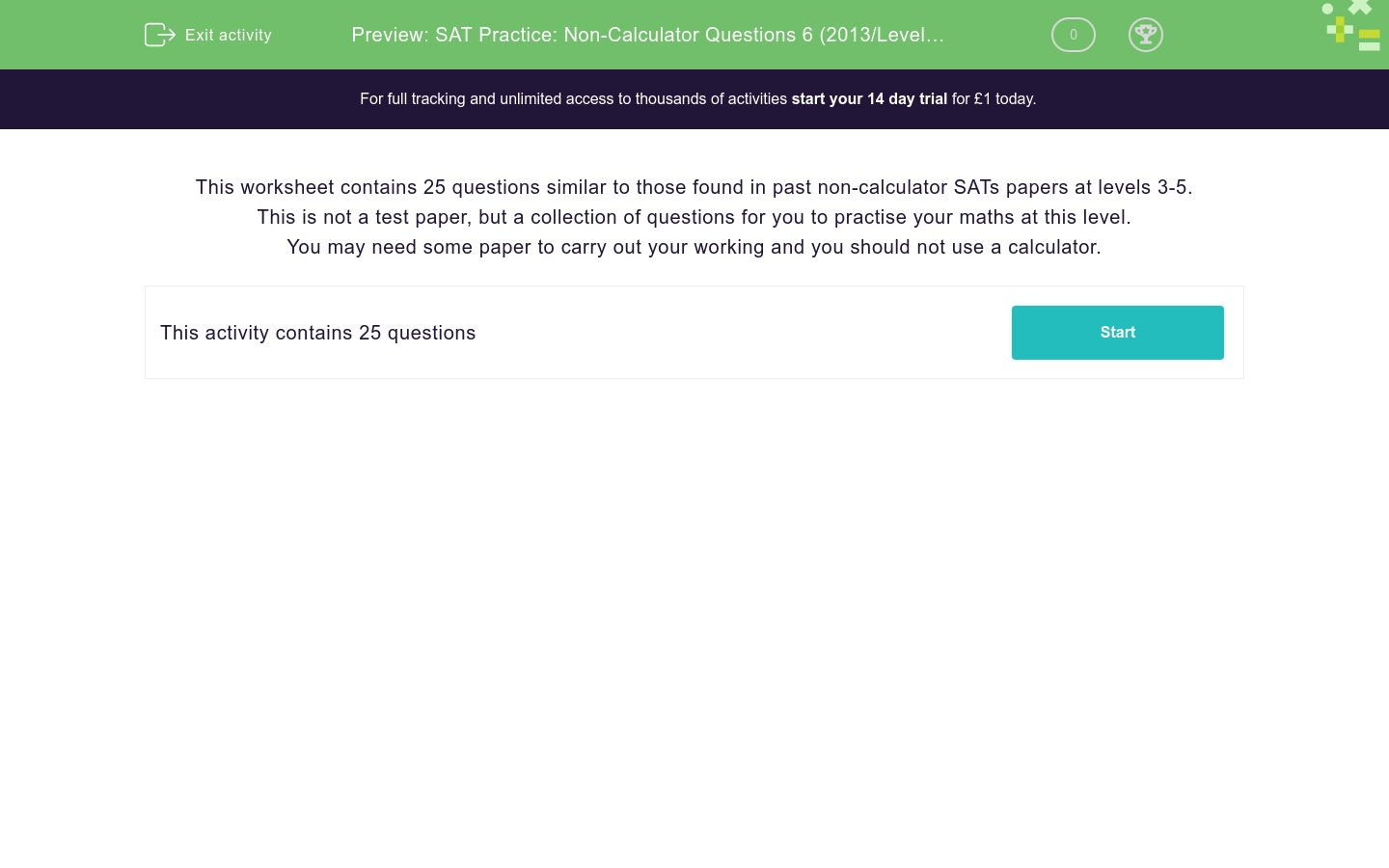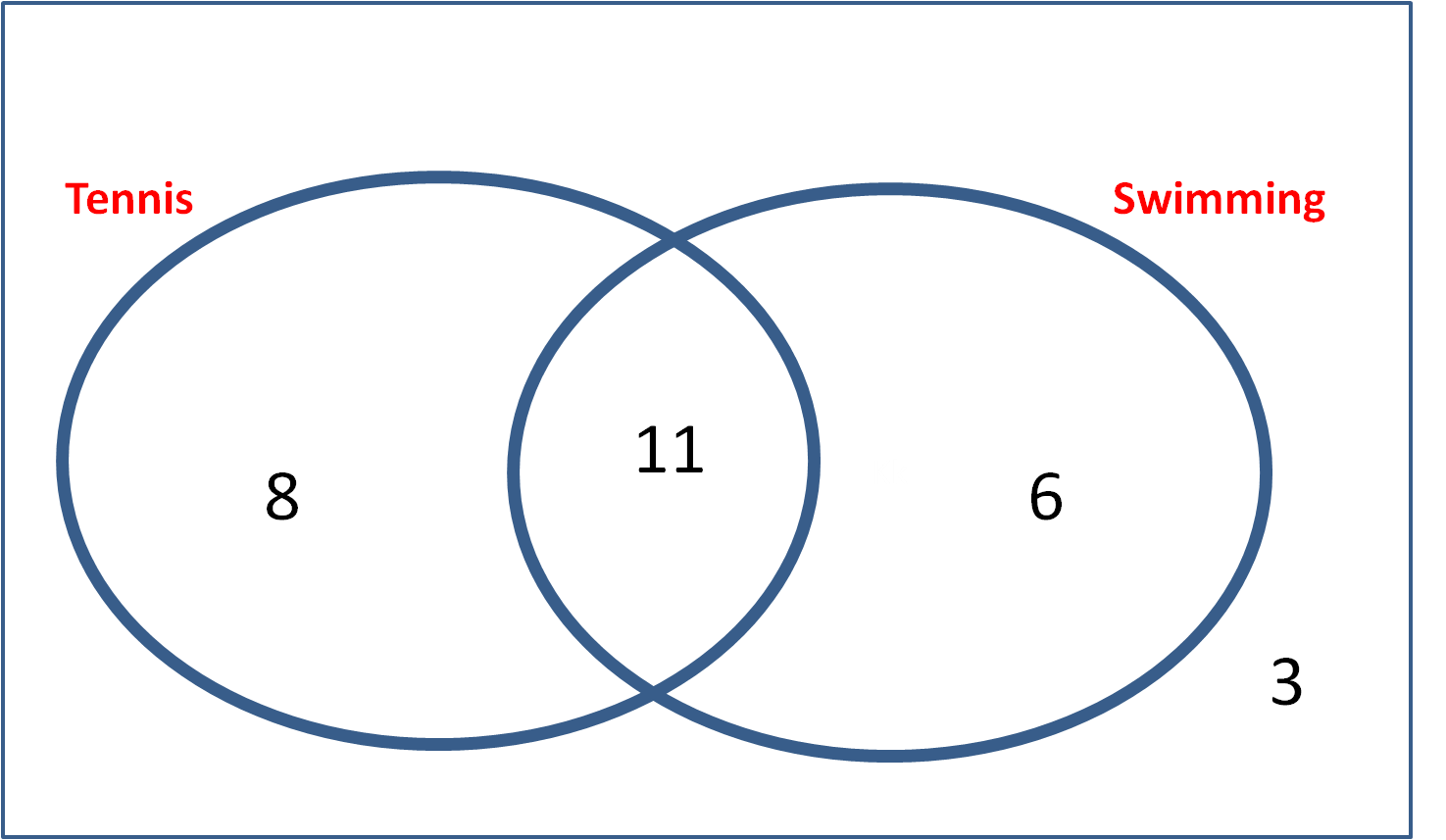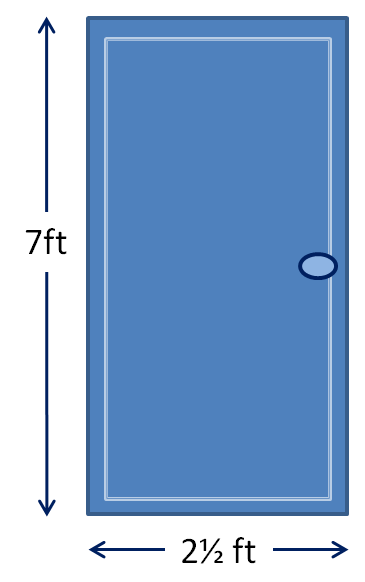# SAT Practice: Non-Calculator Questions 6 (2013/Levels 3-5)

In this worksheet students practise answering questions similar to those found in past non-calculator SATs papers at levels 3-5.Key stage:  KS 2

Curriculum topic:  Practice Papers

Curriculum subtopic:  SAT Non-Calculator

Difficulty level:### QUESTION 1 of 10

This worksheet contains 25 questions similar to those found in past non-calculator SATs papers at levels 3-5.

This is not a test paper, but a collection of questions for you to practise your maths at this level.

You may need some paper to carry out your working and you should not use a calculator.

Select each multiplication which has an answer greater than 150.

12 × 2 × 10

2 × 12 × 3

15 × 10

16 × 5 × 2

16 × 2 × 10

4 × 12 × 3

Envelopes are sold in packs of 15.

Sid needs 72 envelopes.

How many packs must he buy?packs

(Just write the number)

Write the missing number in the calculation below:

7  x=  1288

Write the missing number in the calculation below:-  1209   =  357

Jack has these 10 coins in his pocket.He buys two sweets costing 26p each.

He wishes to use as many coins as possible.

He pays the exact amount.

How many coins does he use to pay for the sweets?Select the calculation which has the greatest answer.

150 ÷ 10

64 ÷ 4

60 ÷ 4

98 ÷ 7

65 ÷ 5

What is the closest square number to 70?

Look at this number line.

To what number is the red arrow pointing?A rectangular window is 1½ metres high.

Its width is 25 cm longer than its height.

How wide is the window in centimetres?cm

(Just write the number)

Four children grow sunflowers in their gardens.

This bar chart shows how high their sunflowers reached in the summer.How much taller is Dev's sunflower than Bert's in centimetres?cm

(Just write the number)

Four children grow sunflowers in their gardens.

This bar chart shows how high their sunflowers reached in the summer.What is the height of Aisha's sunflower to the nearest metre?m

(Just write the number)

Which is greater?

10% of 380 or 20% of 200

10% of 380

20% of 200

The children in a class were asked whether they liked tennis or swimming.

The results are shown in this Venn diagram.How many children did not like tennis?Select all the factors of 60.

1

2

3

4

5

6

8

9

10

12

14

15

20

30

60

Look at this door.On foot (ft) is equivalent to 12 inches.

What is the perimeter of this door?  Give your answer in inches.inches

(Just write the number)

The numbers in this sequence go up 4 each time.

4    8    12    16    ...

The numbers in this sequence go up 5 each time.

5    10    15    20    ...

What is the first number greater than 50 which will be in both sequences?One tennis ball costs £1.45.A sealed pack of 4 tennis balls costs £5.

Jack wants to buy 8 tennis balls.

How much does he save by buying 2 packs instead of 8 individual balls?

£(Just write the number)

From the list of numbers below, select two numbers which add to make 1.02.

0.01

1

3

0.72

0.3

Look at this rectangle, made of smaller squares.What percentage of the whole rectangle has been shaded red?

45%

6%

40%

30%

60%

Look at this pie chart.Calculate the angle that the Apples sector occupies.°

(Just write the number)

Look at this pie chart.What fraction of the whole pie chart is the Bananas section?

1/90

1/4

1/90

1/3

Select the number which is closest to 500.

552

442

449

50

450

Look at the blue shape in the diagram below.It is rotated 90° clockwise around the point X.

On which shape does it land?

A

B

C

Select all the numbers which give 70 when rounded to the nearest 10.

10

65

70

74

75

77

Look at this irregular shape drawn on a square grid.Select all the statements which are TRUE.

EF is at right angles to ED.

EF is parallel to DC.

The angle shown at B is acute.

The angle shown at C is reflex.

AG is parallel to BC.

• Question 1

Select each multiplication which has an answer greater than 150.

12 × 2 × 10
16 × 5 × 2
16 × 2 × 10
EDDIE SAYS
12 × 2 × 10 = 240
2 × 12 × 3 = 72
15 × 10 = 150 which is not greater than 150
16 × 5 × 2 = 160
16 × 2 × 10 = 320
4 × 12 × 3 = 144
• Question 2

Envelopes are sold in packs of 15.

Sid needs 72 envelopes.

How many packs must he buy?packs

(Just write the number)

5
EDDIE SAYS
4 packs gives him 4 × 15 = 60 envelopes.
5 packs gives him 5 × 15 = 75 envelopes.
• Question 3

Write the missing number in the calculation below:

7  x=  1288

184
EDDIE SAYS
Work out 1288 ÷ 7 = 184
• Question 4

Write the missing number in the calculation below:-  1209   =  357

1566
EDDIE SAYS
Work out 357 + 1209 = 1566
• Question 5

Jack has these 10 coins in his pocket.He buys two sweets costing 26p each.

He wishes to use as many coins as possible.

He pays the exact amount.

How many coins does he use to pay for the sweets?8
EDDIE SAYS
He uses two 1p coins, two 5p coins, four 10p coins to make a total of 52p.
• Question 6

Select the calculation which has the greatest answer.

64 ÷ 4
EDDIE SAYS
150 ÷ 10 = 15
64 ÷ 4 = 16
60 ÷ 4 = 15
98 ÷ 7 = 14
65 ÷ 5 = 13
• Question 7

What is the closest square number to 70?

64
EDDIE SAYS
8² = 64 which is 6 less than 70
9² = 81 which is 11 more than 70
• Question 8

Look at this number line.

To what number is the red arrow pointing?0.8
EDDIE SAYS
The marks go up by 0.1 from 0 to 0.1, 0.2, 0.3, etc.
• Question 9

A rectangular window is 1½ metres high.

Its width is 25 cm longer than its height.

How wide is the window in centimetres?cm

(Just write the number)

175
EDDIE SAYS
1½ metres = 150 cm
• Question 10

Four children grow sunflowers in their gardens.

This bar chart shows how high their sunflowers reached in the summer.How much taller is Dev's sunflower than Bert's in centimetres?cm

(Just write the number)

200
EDDIE SAYS
Dev's is 4.5 m = 450 cm tall
Bert's is 2.5 m = 250 cm tall
• Question 11

Four children grow sunflowers in their gardens.

This bar chart shows how high their sunflowers reached in the summer.What is the height of Aisha's sunflower to the nearest metre?m

(Just write the number)

2
EDDIE SAYS
Aisha's sunflower is just over 2 m but less than 2.5 m, so nearer to 2 m than to 3 m.
• Question 12

Which is greater?

10% of 380 or 20% of 200

20% of 200
EDDIE SAYS
10% of 380 = 38
20% of 200 = 200 ÷ 100 × 20 = 40
• Question 13

The children in a class were asked whether they liked tennis or swimming.

The results are shown in this Venn diagram.How many children did not like tennis?9
EDDIE SAYS
6 + 3 = 9
• Question 14

Select all the factors of 60.

1
2
3
4
5
6
10
12
15
20
30
60
EDDIE SAYS
1 × 60
2 × 30
3 × 20
4 × 15
5 × 12
6 × 10
• Question 15

Look at this door.On foot (ft) is equivalent to 12 inches.

What is the perimeter of this door?  Give your answer in inches.inches

(Just write the number)

228
EDDIE SAYS
The perimeter is 7 + 7 + 2½ + 2½ = 14 + 5 = 19 feet.
This is 19 × 12 = 228 inches.
• Question 16

The numbers in this sequence go up 4 each time.

4    8    12    16    ...

The numbers in this sequence go up 5 each time.

5    10    15    20    ...

What is the first number greater than 50 which will be in both sequences?

60
EDDIE SAYS
Multiples of 4 greater than 50 are 52, 56, 60, 64, etc.
Multiples of 5 greater than 50 are 55, 60, 65, etc.
60 is the first number that appears in both sequences.
• Question 17One tennis ball costs £1.45.A sealed pack of 4 tennis balls costs £5.

Jack wants to buy 8 tennis balls.

How much does he save by buying 2 packs instead of 8 individual balls?

£(Just write the number)

1.60
EDDIE SAYS
8 individual balls cost 8 × 1.45 = £11.60
2 packs cost 2 × 5 = £10.00
11.60 - 10.00 = 1.60
• Question 18

From the list of numbers below, select two numbers which add to make 1.02.

0.72
0.3
EDDIE SAYS
0.72 + 0.30 = 1.02
• Question 19

Look at this rectangle, made of smaller squares.What percentage of the whole rectangle has been shaded red?

40%
EDDIE SAYS
6 squares out of 15 is equivalent to:
2 squares out of 5, which is equivalent to:
20 squares out of 50, which is equivalent to:
40 squares out of 100, which is 40%.
• Question 20

Look at this pie chart.Calculate the angle that the Apples sector occupies.°

(Just write the number)

156
EDDIE SAYS
360 - 69 - 45 - 90 = 156
• Question 21

Look at this pie chart.What fraction of the whole pie chart is the Bananas section?

1/4
EDDIE SAYS
90/360 = 9/36 = 1/4
• Question 22

Select the number which is closest to 500.

450
EDDIE SAYS
552 is 52 away
442 is 58 away
449 is 51 away
50 is 450 away
450 is just 50 away
• Question 23

Look at the blue shape in the diagram below.It is rotated 90° clockwise around the point X.

On which shape does it land?

B
EDDIE SAYS
The corner on the point X remains fixed and the rest of the shape is turned a quarter-turn clockwise.
• Question 24

Select all the numbers which give 70 when rounded to the nearest 10.

65
70
74
EDDIE SAYS
10 rounds to 10.
65 rounds up to 10, because it is halfway between 60 and 70 and we always round up in this case.
75 rounds up to 80.
• Question 25

Look at this irregular shape drawn on a square grid.Select all the statements which are TRUE.

EF is parallel to DC.
The angle shown at B is acute.
The angle shown at C is reflex.
EDDIE SAYS
The angle at E is more than 90°.
EF and DC both go one square along and two down.
Acute angles are less than 90° and reflex angles are between 180° and 360°.
AG goes 3 squares along and 1 up, whereas BC goes 2 squares along and 1 up.
---- OR ----

Sign up for a £1 trial so you can track and measure your child's progress on this activity.

### What is EdPlace?

We're your National Curriculum aligned online education content provider helping each child succeed in English, maths and science from year 1 to GCSE. With an EdPlace account you’ll be able to track and measure progress, helping each child achieve their best. We build confidence and attainment by personalising each child’s learning at a level that suits them.